# DAV Class 8 Maths Chapter 12 Brain Teasers Solutions

The DAV Maths Class 8 Solutions and DAV Class 8 Maths Chapter 12 Brain Teasers Solutions of Construction of Quadrilaterals offer comprehensive answers to textbook questions.

## DAV Class 8 Maths Ch 12 Brain Teasers Solutions

Question 1.
Tick (✓) the correct option.
(i) The number of parts required to construct a quadrilateral is
(a) 5
(b) 4
(c) 3
(d) 2
Solution:
To construct a quadrilateral, we shall need a measure of five parts.
Thus, option (a) is the correct answer.

(ii) To construct a parallelogram, the minimum number of dimensions needed is
(a) 2
(b) 3
(c) 4
(d) 1
Solution:
To construct a parallelogram, we shall need a minimum three number of dimensions.
Thus, option (b) is the correct answer.

(iii) The minimum number of dimensions needed to construct a square is
(a) 1
(b) 2
(c) 3
(d) 4
Solution:
We shall need only the side of the square to construct it.
Thus option (a) is the correct answer.(iv) To construct a rectangle, the minimum number of dimensions needed is
(a) 1
(b) 4
(c) 3
(d) 2
Solution:
To construct a rectangle, we shall need only two dimensions.
Thus, option (d) is the correct answer.

(v) Which of the fifth dimensions is needed to construct the given quadrilateral?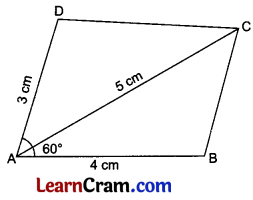(a) BD
(b) ∠C
(c) DC
(d) cannot be constructed
Solution:
To construct the given quadrilateral, we need DC as the fifth dimension.
Thus, option (c) is the correct answer.

Question 2.
(a) Construct a quadrilateral ABCD in which $$\overline{\mathrm{AB}}$$ = $$\overline{\mathrm{CD}}$$ = $$\overline{\mathrm{BC}}$$ = $$\overline{\mathrm{AD}}$$ = 5 cm and diagonal $$\overline{\mathrm{AC}}$$ = 6.5 cm.
Solution:
Steps of Construction:
1. Draw $$\overline{\mathrm{AB}}$$ = 5 cm.
2. Draw arcs from A and B with radii 6.5 cm and 5 cm respectively to meet at C.
3. Join $$\overline{\mathrm{AC}}$$ and $$\overline{\mathrm{BC}}$$.
4. Draw arcs from A and C with a radius of 5 cm each to meet at D.
5. Join $$\overline{\mathrm{CD}}$$ and $$\overline{\mathrm{DA}}$$.
6. ABCD is the required quadrilateral.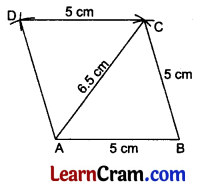(b) Construct a quadrilateral PQRS in which $$\overline{\mathrm{PQ}}$$ = $$\overline{\mathrm{QR}}$$ = $$\overline{\mathrm{RS}}$$ = 5 cm, diagonals $$\overline{\mathrm{PR}}$$ = $$\overline{\mathrm{QS}}$$ = 7.5 cm.
Solution:
Steps of Construction:
1. Draw $$\overline{\mathrm{PQ}}$$ = 5 cm.
2. Draw arcs from P and Q with radii 7.5 cm and 5 cm respectively to meet at R.
3. Join $$\overline{\mathrm{PR}}$$ and $$\overline{\mathrm{QR}}$$.
4. Draw arcs from Q and R with radii 7.5 cm and 5 cm respectively to meet at S.
5. Join $$\overline{\mathrm{RS}}$$, $$\overline{\mathrm{QS}}$$ and $$\overline{\mathrm{QP}}$$.
6. PQRS is the required quadrilateral.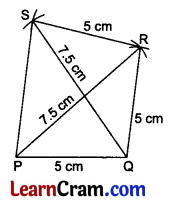(c) Construct a quadrilateral ABCD in which $$\overline{\mathrm{AB}}$$ = $$\overline{\mathrm{CD}}$$ = 5 cm, $$\overline{\mathrm{BC}}$$ = 4 cm, diagonals $$\overline{\mathrm{AC}}$$ = 9 cm, BD = 8 cm.
Solution:
Steps of Construction:
$$\overline{\mathrm{AB}}$$ = 5 cm, $$\overline{\mathrm{BC}}$$ = 4 cm and $$\overline{\mathrm{AC}}$$ = 9 cm.
∴ $$\overline{\mathrm{AB}}+\overline{\mathrm{BC}}=\overline{\mathrm{AC}}$$
5 + 4 = 9
9 cm = 9 cm
Point C will lie on AB produced.
Hence quadrilateral ABCD is not possible to form.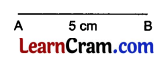(d) Construct a quadrilateral ABCD in which $$\overline{\mathrm{AB}}$$ = $$\overline{\mathrm{BC}}$$ = $$\overline{\mathrm{CD}}$$ = $$\overline{\mathrm{DA}}$$ = 5 cm and ∠A = 120°. What type of quadrilateral is it?
Solution:
Steps of Construction:
1. Draw $$\overline{\mathrm{AB}}$$ = 5 cm.
2. Draw ∠A = 120° and cut $$\overline{\mathrm{AD}}$$ = 5 cm.
3. Draw two arcs at B and D with a radius of 5 cm each to meet at C.
4. Join $$\overline{\mathrm{BC}}$$ and $$\overline{\mathrm{DC}}$$.
5. ABCD is the required quadrilateral.
6. The constructed quadrilateral is a rhombus.(e) Construct a quadrilateral PQRS in which $$\overline{\mathrm{PQ}}$$ = $$\overline{\mathrm{RQ}}$$ = 4 cm, and ∠P = ∠Q = ∠R = ∠S = 90°. What type of quadrilateral is it?
Solution:
Steps of Construction:
1. Draw $$\overline{\mathrm{PQ}}$$ = 4 cm.
2. Draw ∠Q = 90° and cut $$\overline{\mathrm{QR}}$$ = 4 cm.
3. Draw arcs from P and R with a radius of 4 cm each to meet at S.
4. Join $$\overline{\mathrm{RS}}$$ and $$\overline{\mathrm{PS}}$$.
5. PQRS is the required quadrilateral.
6. PQRS is a square.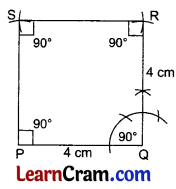(f) Construct a quadrilateral ABCD in which $$\overline{\mathrm{AB}}$$ = $$\overline{\mathrm{BC}}$$ = $$\overline{\mathrm{CD}}$$ = $$\overline{\mathrm{AD}}$$ = 5 cm, diagonal $$\overline{\mathrm{AC}}$$ = 7 cm and diagonals bisect each other at right angles. What type of quadrilateral is it?
Solution:
Steps of Construction:
1. Draw $$\overline{\mathrm{AB}}$$ = 5 cm.
2. Draw arcs from A and B with radii 7 cm and 5 cm respectively to meet at C.
3. Draw the right bisector of $$\overline{\mathrm{AC}}$$ at O.
4. Make $$\overline{\mathrm{CD}}$$ = 5 cm.
5. Join D to A.
6. ABCD is the required quadrilateral.
7. The construct quadrilateral ABCD is a rhombus.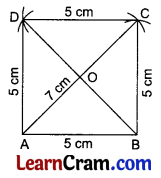(g) Construct a quadrilateral ABCD in which $$\overline{\mathrm{AB}}$$ = $$\overline{\mathrm{CD}}$$ = 3 cm, $$\overline{\mathrm{BC}}$$ = $$\overline{\mathrm{AD}}$$ = 4 cm and diagonals are 5 cm each. What type of quadrilateral is it?
Solution:
Steps of Construction:
1. Draw $$\overline{\mathrm{AB}}$$ = 3 cm.
2. Draw arcs from A and B with radii 5 cm and 4 cm respectively to meet at C.
3. Join $$\overline{\mathrm{AC}}$$ and $$\overline{\mathrm{BC}}$$.
4. Draw arcs at A and C with radius 4 cm and 3 cm respectively to meet at D.
5. Join $$\overline{\mathrm{AD}}$$ and $$\overline{\mathrm{CD}}$$.
6. ABCD is the required quadrilateral.
7. The construct quadrilateral ABCD is a rectangle.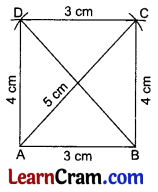(h) Construct a quadrilateral ABCD in which all sides are 5 cm each and ∠A = ∠B = 90°.
Solution:
Steps of Construction:
1. Draw $$\overline{\mathrm{AB}}$$ = 5 cm.
2. Draw ∠A = ∠B = 90°.
3. Make $$\overline{\mathrm{BC}}$$ = $$\overline{\mathrm{AD}}$$ = 5 cm.
4. Join $$\overline{\mathrm{CD}}$$.
5. ABCD is the required quadrilateral.### DAV Class 8 Maths Chapter 12 HOTS

Question 1.
Construct a trapezium ABCD with AB || CD where AB = 6 cm, CD = 4 cm, ∠A = 70°, ∠ABD = 30°.
Solution:
Steps of Construction:
1. Draw $$\overline{\mathrm{AB}}$$ = 6 cm.
2. At A, construct ∠BAX = 70°.
3. At B, construct ∠ABY = 30°. Arm $$\overrightarrow{\mathrm{BY}}$$ of ∠ABY interesect $$\overrightarrow{\mathrm{AX}}$$. Name the point of intersection as D.
4. Since interior angles of the same side of transversal (AD) of parallel lines (AB || CD) are supplementary. So, ∠ADZ = 180° – 70°= 110°. Draw ∠ADZ = 110° at D.
5. Cut off $$\overline{\mathrm{DC}}$$ = 4 cm on ray DZ.
6. Join B to C.
7. ABCD is the required trapezium.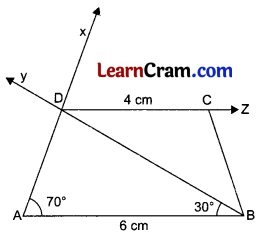Question 2.
Construct a rectangle whose length is twice its width and whose perimeter is equal to the perimeter of a rhombus of side 6 cm.
Solution:
To construct the rectangle, we need its length and width.
Let the width of the rectangle be x cm.
Then, the length of the rectangle = 2x
According to the question,
Perimeter of the rectangle = Perimeter of rhombus
⇒ 2(length + width) = 4 × side
⇒ 2(2x + x) = 4 × 6
⇒ 2 × 3x = 24
⇒ 6x = 24
⇒ x = 4
∴ Length of the rectangle = 8 cm and width = 4 cm.

Steps of Construction:
1. Draw the line segment $$\overline{\mathrm{AB}}$$ = 8 cm
2. At A and B, construct ∠BAX = 90° and ∠ABY = 90° respectively.
3. Cut off $$\overline{\mathrm{AD}}$$ = 4 cm on ray AX and $$\overline{\mathrm{BC}}$$ = 4 cm on ray BY.
4. Join C to D.
5. ABCD is the required rectangle.### DAV Class 8 Maths Chapter 12 Enrichment Questions

Question 1.
Draw an equilateral triangle ABD of side 4.5 cm. Taking one of its sides as a diagonal, complete the rhombus ABCD.
Solution:
Steps of Construction:
1. Draw line segment $$\overline{\mathrm{AB}}$$ = 4.5 cm.
2. Taking A and B as centres and a radius of 4.5 cm each time, draw two arcs that intersect each other at D.
3. Join A to D and B to D. ΔABD is an equilateral triangle.
Note: Two sides of rhombus ABCD i.e., AB and AD, and one diagonal BD are given here. We know that diagonals of rhombus bisect each other at right angles.
4. Draw a perpendicular bisector (AOX) of diagonal BD which meets AB at A.
5. Cut off $$\overline{\mathrm{OC}}$$ = $$\overline{\mathrm{OA}}$$.
6. Join D to C and B to C.
7. ABCD is the required rhombus.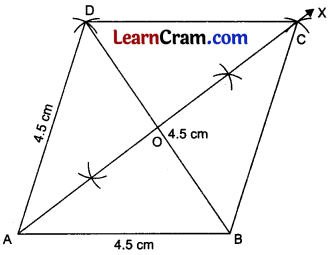Question 2.
Construct a rhombus of perimeter 20 cm and one base angle the supplement of 130°.
Solution:
The perimeter of the rhombus = 20 cm (given)
⇒ 4 × side = 20 cm
⇒ side = 5 cm
Let one base angle of the rhombus be x.
Supplement of x = 130° (given)
⇒ 180° -x = 130 (∵ x + 130° = 180°)
⇒ x = 50°
We have one angle of rhombus = 50° and one side = 5 cm.

Steps of Construction:
1. Draw a line segment AB =5 cm
2. Draw ∠BAX = 50° at A.
3. Cut off AD = 5 cm.
4. Taking radius = 5 cm, draw arcs from D and B which cut each other at C.
5. Join D to C and B to C.
6. ABCD is the required rhombus.Question 1.
Construct a quadrilateral PQRS in which $$\overline{\mathrm{PQ}}$$ = 4 cm, $$\overline{\mathrm{QR}}$$ = 5.5 cm, $$\overline{\mathrm{RS}}$$ = 5 cm, ∠Q = 60° and ∠R = 75°.
Solution:
Steps of Construction:
1. Draw $$\overline{\mathrm{PQ}}$$ = 4 cm.
2. Draw ∠Q = 60°.
3. Make $$\overline{\mathrm{QR}}$$ = 5.5 cm.
4. Draw ∠R = 75°.
5. Cut $$\overline{\mathrm{RS}}$$ = 5 cm.
6. Join S to P.
7. PQRS is the required quadrilateral.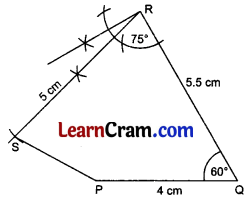Question 2.
Construct the following quadrilateral if possible:
$$\overline{\mathrm{AB}}$$ = 4 cm, $$\overline{\mathrm{BC}}$$ = 4.5 cm, $$\overline{\mathrm{CD}}$$ = 4 cm and the diagonal $$\overline{\mathrm{AC}}$$ = 9 cm.
Solution:
Steps of Construction:
1. Draw $$\overline{\mathrm{AB}}$$ = 4 cm.
2. Draw arcs from A and B with radii of 9 cm and 4.5 cm respectively.
3. The two arcs do not cut each other anywhere
Reason: For a triangle ABC
$$\overline{\mathrm{AB}}+\overline{\mathrm{BC}}>\overline{\mathrm{AC}}$$
But 4 cm + 4.5 cm < 9 cm
⇒ 8.5 cm < 9 cm
So, the construction of quadrilateral ABCD is not possible.Question 3.
Construct a quadrilateral PQRS where $$\overline{\mathrm{PQ}}$$ = 4 cm, $$\overline{\mathrm{QR}}$$ = 6 cm, $$\overline{\mathrm{RS}}$$ = 5 cm, $$\overline{\mathrm{PS}}$$ = 5.5 cm and $$\overline{\mathrm{PR}}$$ = 7 cm.
Solution:
Steps of Construction:
1. Draw $$\overline{\mathrm{PQ}}$$ = 4 cm.
2. Draw two arcs from P and Q with radii 7 cm and 6 cm respectively to meet at R.
3. Join $$\overline{\mathrm{PR}}$$ and $$\overline{\mathrm{QR}}$$.
4. Draw two arcs from P and R with radii 5.5 cm and 5 cm respectively to meet at S.
5. Join $$\overline{\mathrm{PS}}$$ and $$\overline{\mathrm{RS}}$$.
7. PQRS is the required quadrilateral.Question 4.
Construct a MIST where MI = 3.5 cm, IS = 6.5 cm, ∠M = 75°, ∠I = 105° and ∠S = 120°.
Solution:
Steps of Construction:
1. Draw $$\overline{\mathrm{MI}}$$ = 3.5 cm.
2. Draw ∠M = 75° and ∠I = 105°.
3. Cut $$\overline{\mathrm{IS}}$$ = 6.5 cm.
4. Draw ∠S = 120°.
5. The ray of ∠S and the ray of ∠M intersect each other at T.
6. MIST is the required quadrilateral.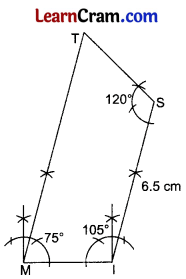Question 5.
Construct a quadrilateral ABCD where $$\overline{\mathrm{AB}}$$ = 4 cm, $$\overline{\mathrm{BC}}$$ = 5 cm, $$\overline{\mathrm{CD}}$$ = 6.5 cm and ∠B = 105° and ∠C = 80°.
Solution:
Steps of Construction:
1. Draw $$\overline{\mathrm{AB}}$$ = 4 cm.
2. Draw ∠B = 105° and make $$\overline{\mathrm{BC}}$$ = 5 cm.
3. Draw ∠C = 80° and make $$\overline{\mathrm{CD}}$$ = 6.5 cm.
4. Join D to A.
5. ABCD is the required quadrilateral.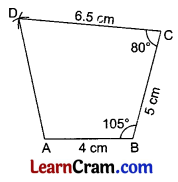Question 6.
Construct a quadrilateral TRUE in which TR = 3.5 cm, RU = 3 cm, UE = 4 cm, ∠R = 75° and ∠U = 120°.
Solution:
Steps of Construction:
1. Draw TR 3.5 cm.
2. Draw ∠R = 75° and cut $$\overline{\mathrm{RU}}$$ = 4 cm.
3. Draw ∠U = 120° and cut $$\overline{\mathrm{UE}}$$ = 4 cm.
4. Join E to T.
5. TRUE is the required quadrilateral.